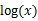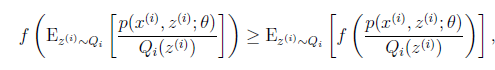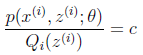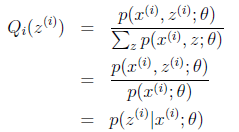# 1. 通过一个简单的例子直观上理解EM的核心思想

## 0x1: 问题背景

Coin_a：P1；1-P1
Coin_b：P2；1-P2

Coin_a 正 正 反 正 反 3正-2反
Coin_b 反 反 正 正 反 2正-3反
Coin_a 正 反 反 反 反 1正-4反
Coin_b 正 反 反 正 正 3正-2反
Coin_a 反 正 正 反 反 2正-3反

P1 = （3+1+2）/ 15 = 0.4
P2= （2+3）/10 = 0.5

## 0x2: 加入隐变量

Unknown 正 正 反 正 反 3正-2反
Unknown 反 反 正 正 反 2正-3反
Unknown 正 反 反 反 反 1正-4反
Unknown 正 反 反 正 正 3正-2反
Unknown 反 正 正 反 反 2正-3反

我们先不管三七二十一，在所有可行解空间中随机初始化一个P1、P2，基于初始化的P1、P2来估计z，然后基于z按照最大似然概率法则去估计新的P1、P2
（1）如果新的P1、P2和我们初始化的初始值差距小于一个阈值，说明收敛了，我们初始化的P1、P2有很大可能就是真实值
（2）如果新的P1、P2和我们初始化的初始值差距很大，则继续用新的P1、P2进行迭代，重复本轮步骤，直至收敛

## 0x3: EM初级版

Coin_a P1 = 0.1; 1-P1 = 0.9
Coin_b P2 = 0.8; 1-P2 = 0.2

1 0.1 * 0.1 * 0.1 * 0.9 * 0.9 = 0.00081（3正2反） 0.8 * 0.8 * 0.8 * 0.2 * 0.2 = 0.02048（3正2反） Coin_b
2 0.1 * 0.1 * 0.9 * 0.9 * 0.9 = 0.00729（2正3反） 0.8 * 0.8 * 0.2 * 0.2 * 0.2 = 0.00512（2正3反） Coin_a
3 0.1 * 0.9 * 0.9 * 0.9 * 0.9（1正4反） 0.8 * 0.2 * 0.2 * 0.2 * 0.2 = 0.00512（1正4反） Coin_a
4 0.1 * 0.1 * 0.1 * 0.9 * 0.9（3正2反） 0.8 * 0.8 * 0.8 * 0.2 * 0.2 = 0.02048（3正2反） Coin_b
5 0.1 * 0.1 * 0.9 * 0.9 * 0.9 = 0.00729（2正3反） 0.8 * 0.8 * 0.2 * 0.2 * 0.2 = 0.00512（2正3反） Coin_a

Coin_a P1 = （2 + 1 + 2）/ 15 = 0.33333：抛了3轮，每轮5次，总计15次
Coin_b P1 = （3 + 3）/ 10 = 0.6：抛了2轮，每轮5次，总计10次

Coin_a P1Coin_b P2

1. 为啥新估计出的P1、P2会更加接近真实值？只是本例的偶然吗？答案是否定的，我们接下来的章节会介绍理论数学推导来证明EM的单调性
2. 不管我们如何初始化，迭代一定会收敛到真实的P1、P2吗？答案是否定的，这取决于我们的P1、P2初始化值，EM对初始值比较敏感，因为它很容易陷入到局部最优

## 0x4: EM进阶版

1 0.1 * 0.1 * 0.1 * 0.9 * 0.9 = 0.00081（3正2反） 0.8 * 0.8 * 0.8 * 0.2 * 0.2 = 0.02048（3正2反）
2 0.1 * 0.1 * 0.9 * 0.9 * 0.9 = 0.00729（2正3反） 0.8 * 0.8 * 0.2 * 0.2 * 0.2 = 0.00512（2正3反）
3 0.1 * 0.9 * 0.9 * 0.9 * 0.9 = 0.06561（1正4反） 0.8 * 0.2 * 0.2 * 0.2 * 0.2 = 0.00128（1正4反）
4 0.1 * 0.1 * 0.1 * 0.9 * 0.9 = 0.00081（3正2反） 0.8 * 0.8 * 0.8 * 0.2 * 0.2 = 0.02048（3正2反）
5 0.1 * 0.1 * 0.9 * 0.9 * 0.9 = 0.00729（2正3反） 0.8 * 0.8 * 0.2 * 0.2 * 0.2 = 0.00512（2正3反）

1 0.00081 / (0.00081 + 0.02048) = 0.0380460310005 0.961953969
2 0.00729 / (0.00729 + 0.00512) = 0.587429492345 0.412570507655
3 0.06561/ (0.06561+ 0.00128) = 0.980864105247 0.0191358947526
4 0.00081 / (0.00081 + 0.02048) = 0.0380460310005 0.961953969
5 0.00729 / (0.00729 + 0.00512) = 0.587429492345 0.412570507655

Unknown 正 正 反 正 反 3正-2反
Unknown 反 反 正 正 反 2正-3反
Unknown 正 反 反 反 反 1正-4反
Unknown 正 反 反 正 正 3正-2反
Unknown 反 正 正 反 反 2正-3反

1 0.0380460310005 * 3 = 0.114138093001 0.0380460310005 * 2 = 0.076092062001 0.961953969 * 3 = 2.885861907 0.961953969 * 2 = 1.923907938
2 0.587429492345 * 2 = 1.17485898469 0.587429492345 * 3 = 1.76228847703 0.412570507655 * 2 = 0.82514101531 0.412570507655 * 3 = 1.23771152296
3 0.980864105247 * 1 = 0.980864105247 0.980864105247 * 4 = 3.92345642099 0.0191358947526 * 1 = 0.0191358947526 0.0191358947526 * 4 = 0.0765435790104
4 0.114138093001 0.076092062001 2.885861907 1.923907938
5 1.17485898469 1.76228847703 0.82514101531 1.23771152296

Coin_a P1 = 3.55885826063 / (3.55885826063 + 7.60021749905) = 0.318920521491
Coin_b P1 = 7.44114173937 / (7.44114173937 + 6.39978250093) = 0.53761884757

Coin_a P1Coin_b P2

EM初级版估计出的概率值 0.3333 0.6
EM高级版估计出的概率值 0.318920521491 0.537618847571. 初始的第一步是原始的最大似然估计要求的参数，我们通过随机初始化的方式赋予了一个随机初始值
2. Expectation这一步的目的是估计出隐变量的概率分布
3. Maximization这一步就是原始的最大似然概率估计（基于本轮Expectation得到的隐变量概率分布），估计出模型的参数

https://www.zhihu.com/question/27976634
http://blog.sina.com.cn/s/blog_a7da5cda010158b3.html
http://www.cnblogs.com/Gabby/p/5344658.html
http://blog.csdn.net/livecoldsun/article/details/40833829

# 2. EM算法的引入

EM算法是一种迭代算法，1977年由Dempster等人总结提出，用于含有隐变量（hidden variable）的概率模型参数的极大似然估计，或极大后验概率估计。EM算法的每轮迭代由两步组成：E步（求期望 expectation）；M步（求极大似然），所以这一算法称为期望极大算法（expectation maximization algorithm EM算法）

## 0x1: EM算法数学定义

（1）随机初始化模型参数的初值，开始迭代。模型参数的初值可以任意随机选择，但需要注意EM算法对初值很敏感（EM每轮迭代都是在求局部最优，并不能保证最后得到全局最优解）

（2）E步：为第 i 次迭代参数的估计值，在第 i + 1 次迭代的E步，计算：，这里，是在给定观测数据Y和当前的参数估计下隐变量数据Z的条件概率分布。E步求Q函数，Q函数式中Z是未观测数据，Y是观测数据，可以看到，Q函数是为M步进行最大似然估计做数学公式解析准备的，每轮迭代实际上是在求Q函数机器极大。即EM的迭代是不断调整隐变量的极大似然值，使其（隐变量）接近真实值，可以预见，只有隐变量越接近真实值，模型参数的极大似然才能随着不断接近真实值

（3）M步：求使极大化的（模型参数），确定第 i + 1次迭代的参数的估计值。每次迭代使模型参数的似然函数增加或达到局部极值

（4）重复（2）和（3）步，直至收敛，收敛停止条件一般是对较小的正数，若满足（及本轮相较于上一轮的优化幅度小于一定阈值）则停止迭代

## 0x2: 举一个实际的例子说明EM算法的推导当且仅当X是常数的时候等号成立，如果f（X）是凹函数，不等号反向

log函数是凹函数，所以E（f(x)） <= f（E(x)）1. 首先我们初始化一个θ1，根据它求似然函数一个紧的下界，也就是图中第一条黑短线，黑短线上的值虽然都小于似然函数的值，但至少有一点可以满足等号（所以称为紧下界），最大化小黑短线我们就hit到至少与似然函数刚好相等的位置，对应的横坐标就是我们的新的θ2
2. 如此进行，只要保证随着θ的更新，每次最大化的小黑短线（Jensen不等式的下界）值都比上次的更大，那么算法收敛，最后就能最大化到似然函数的极大值处1）到（2）比较直接，就是分子分母同乘以一个相等的函数。（2）到（3）利用了Jensen不等式，考虑到是凹函数（二阶导数小于0），而且循环重复直到收敛 {       （E步）对于每一个i，计算       （M步）计算

http://blog.csdn.net/yzheately/article/details/51164441
http://www.cnblogs.com/yymn/p/4769736.html
http://blog.csdn.net/xiaozezepingping/article/details/29849007
http://blog.csdn.net/livecoldsun/article/details/40833829
http://www.cnblogs.com/Gabby/p/5344658.html

# 3. EM算法的收敛性

## 0x1: 定理1 - EM对模型参数的最大似然估计是单调递增的为观测数据的似然函数，为EM算法得到的参数估计序列（每轮迭代的最大似然参数估计结果），为对应的似然函数序列，则是单调递增的，即，下面证明这一点

（1）上式等号右边第一项，由于使达到极大，所以有（2）上式等号右边第二项展开得：1. 等号由Jensen不等式得到
2. 最后一步等于0是因为Z是已知的，概率为1，log = 0
3. 倒数第二步的分母约分根据条件概率边缘分布原理

http://blog.csdn.net/xiaozezepingping/article/details/29849007
http://blog.csdn.net/yzheately/article/details/51164441
http://www.cnblogs.com/yymn/p/4769736.html

# 4. EM算法在高斯混合模型（GMM）学习中的应用

EM算法的一个重要应用是高斯混合模型的参数估计，高斯混合模型应用广泛，在许多情况下，EM算法是学习高斯混合模型（Gaussian misture model）的有效方法

## 0x2: 高斯混合模型参数估计中的EM的算法

### 1. 明确隐变量，写出完全数据的对数似然函数

（1）首先按照概率选择第 k 个高斯分布模型（2）然后依照第 k 个分模型的概率分布生成观测数据。这是观测数据，是已知的；

（3）反映观测数据来自第 k 个分模型的数据是未知的（隐变量），，以隐变量表示，其定义如下：，其中是0-1随机变量### 2. EM算法的E步：确定Q函数是在当前模型参数下第 j  个观测数据来自第 k 个分模型的概率，称为分模型 k 对观测数据的响应度代入上式得：### 3. 确定EM算法的M步

（1）分别对求偏导数并令其为0：（2）求是在条件下求偏导数并令其为0得到的：，可以看到，对隐变量其实就是在进行最大似然估计

http://www.cnblogs.com/xingshansi/p/6584555.html
http://www.cnblogs.com/mindpuzzle/archive/2013/04/24/3036447.html

# 5. EM算法在思想上和K-means的异同点

## 0x1: 简单回顾K-means算法

k-means算法是一种得到最广泛使用的聚类算法。 它是将各个聚类子集内的所有数据样本的均值作为该聚类的代表点。k-means 计算过程：

1. 随机选择k个类簇的中心
2. 计算每一个样本点到所有类簇中心的距离，选择最小距离作为该样本的类簇
3. 重新计算所有类簇的中心坐标，直到达到某种停止条件(迭代次数/簇中心收敛/最小平方误差)

## 0x2: K-means算法是EM算法的一个特例数学证明K-means的这种方法相当于进行了"硬分类"，每个样本点有且只能在一个类别Z中，而不能具有概率分布

E步骤 - 根据当前质心求样本的分类最大似然结果Zi

1、计算，以及（联合概率），这里使用正比于符号是考虑到距离点最近的类中心可能不止一个，下面推导假设类中心点只有一个，并且该类中心的编号为2、计算目标函数EM算法中E步对隐变量 Zi 求其最大似然值，这等价于K-means算法中对于每一个点找当前最近的聚类中心M步骤 - 重新寻找新的质心：

## 0x3: k-means算法与由EM算法推导出的的关系

1. k-means是两个步骤交替进行，可以分别看成E步和M步；
2. M步中将每类的中心更新为分给该类各点的均值，可以认为是在「各类分布均为单位方差的高斯分布」的假设下，最大化似然值；
3. E步中将每个点分给中心距它最近的类（硬分配），可以看成是EM算法中E步（软分配）的近似。

k-means对GMM的简化有

•，混合权重相等
•，各个分模型的协方差相等，且固定为单位矩阵的倍数
•分配给各个分模型的方式，由基于概率分布变为winner-take-all方式的 hard 赋值

http://www.cnblogs.com/arachis/p/KMeans.html
https://www.zhihu.com/question/49972233
http://www.cnblogs.com/mindpuzzle/archive/2013/04/24/3036447.html

# 6. EM算法在高斯混合模型(GMM)学习中的应用

EM算法的一个重要应用是高斯混合模型的参数估计

## 0x1: 利用GMM进行无监督聚类

# -*- coding:utf-8 -*-

import matplotlib as mpl
import matplotlib.pyplot as plt

import numpy as np

from sklearn import datasets
from sklearn.mixture import GaussianMixture
from sklearn.model_selection import StratifiedKFold

print(__doc__)

colors = ['navy', 'turquoise', 'darkorange']

def make_ellipses(gmm, ax):
for n, color in enumerate(colors):
if gmm.covariance_type == 'full':
covariances = gmm.covariances_[n][:2, :2]
elif gmm.covariance_type == 'tied':
covariances = gmm.covariances_[:2, :2]
elif gmm.covariance_type == 'diag':
covariances = np.diag(gmm.covariances_[n][:2])
elif gmm.covariance_type == 'spherical':
covariances = np.eye(gmm.means_.shape) * gmm.covariances_[n]
v, w = np.linalg.eigh(covariances)
u = w / np.linalg.norm(w)
angle = np.arctan2(u, u)
angle = 180 * angle / np.pi  # convert to degrees
v = 2. * np.sqrt(2.) * np.sqrt(v)
ell = mpl.patches.Ellipse(gmm.means_[n, :2], v, v,
180 + angle, color=color)
ell.set_clip_box(ax.bbox)
ell.set_alpha(0.5)

# Break up the dataset into non-overlapping training (75%) and testing
# (25%) sets.
skf = StratifiedKFold(n_splits=4)
# Only take the first fold.
train_index, test_index = next(iter(skf.split(iris.data, iris.target)))

X_train = iris.data[train_index]
y_train = iris.target[train_index]
X_test = iris.data[test_index]
y_test = iris.target[test_index]

n_classes = len(np.unique(y_train))

# Try GMMs using different types of covariances.
estimators = dict((cov_type, GaussianMixture(n_components=n_classes,
covariance_type=cov_type, max_iter=20, random_state=0))
for cov_type in ['spherical', 'diag', 'tied', 'full'])

n_estimators = len(estimators)

plt.figure(figsize=(3 * n_estimators // 2, 6))
left=.01, right=.99)

for index, (name, estimator) in enumerate(estimators.items()):
# Since we have class labels for the training data, we can
# initialize the GMM parameters in a supervised manner.
estimator.means_init = np.array([X_train[y_train == i].mean(axis=0)
for i in range(n_classes)])

# Train the other parameters using the EM algorithm.
estimator.fit(X_train)

h = plt.subplot(2, n_estimators // 2, index + 1)
make_ellipses(estimator, h)

for n, color in enumerate(colors):
data = iris.data[iris.target == n]
plt.scatter(data[:, 0], data[:, 1], s=0.8, color=color,
label=iris.target_names[n])
# Plot the test data with crosses
for n, color in enumerate(colors):
data = X_test[y_test == n]
plt.scatter(data[:, 0], data[:, 1], marker='x', color=color)

y_train_pred = estimator.predict(X_train)
train_accuracy = np.mean(y_train_pred.ravel() == y_train.ravel()) * 100
plt.text(0.05, 0.9, 'Train accuracy: %.1f' % train_accuracy,
transform=h.transAxes)

y_test_pred = estimator.predict(X_test)
test_accuracy = np.mean(y_test_pred.ravel() == y_test.ravel()) * 100
plt.text(0.05, 0.8, 'Test accuracy: %.1f' % test_accuracy,
transform=h.transAxes)

plt.xticks(())
plt.yticks(())
plt.title(name)

plt.legend(scatterpoints=1, loc='lower right', prop=dict(size=12))

plt.show()http://scikit-learn.org/stable/modules/generated/sklearn.mixture.GMM.html